# Stirling's Approximation for n!

When evaluating distribution functions for statistics, it is often necessary to evaluate the factorials of sizable numbers, as in the binomial distribution: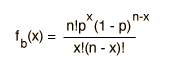A helpful and commonly used approximate relationship for the evaluation of the factorials of large numbers is Stirling's approximation: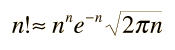A slightly more accurate approximation is the following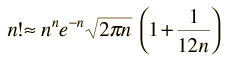but in most cases the difference is small. This additional term does give a way to assess whether the approximation has a large error.

Stirling's approximation is also useful for approximating the log of a factorial, which finds application in evaluation of entropy in terms of multiplicity, as in the Einstein solid. The log of n! is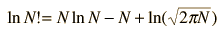but the last term may usually be neglected so that a working approximation is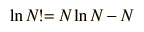Shroeder gives a numerical evaluation of the accuracy of the approximations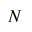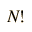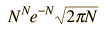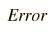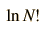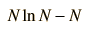1 1 .922 7.7% 0 -1 ... 10 3628800 3598696 .83% 15.1 13.0 13.8% 100 9 x 10157 9 x 10157 .083% 364 360 .89%
 Relation to Gamma Function
Index

Statistics concepts

Reference
Schroeder
App. B
Ch 2

 HyperPhysics*****HyperMath R Nave
Go Back